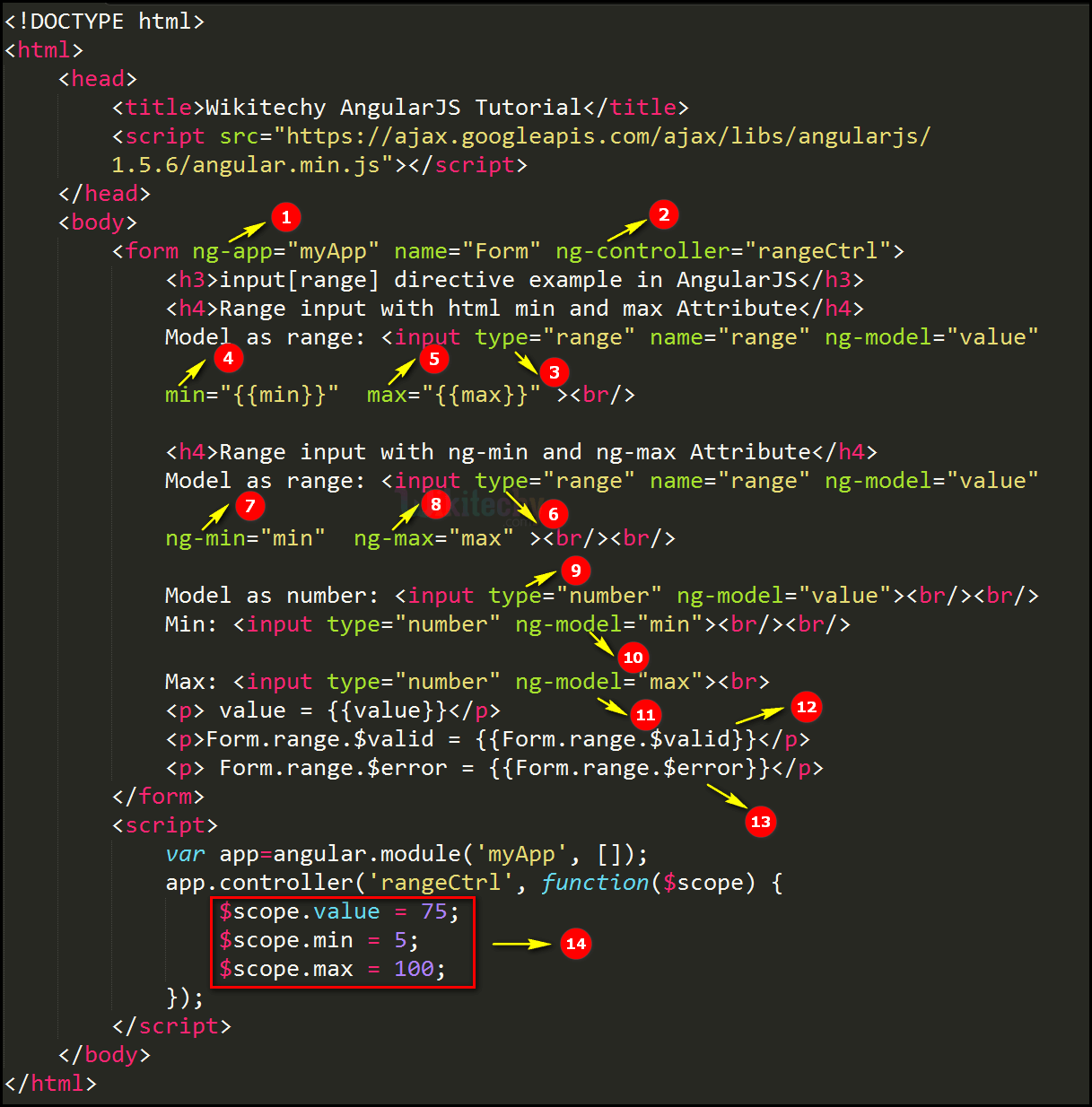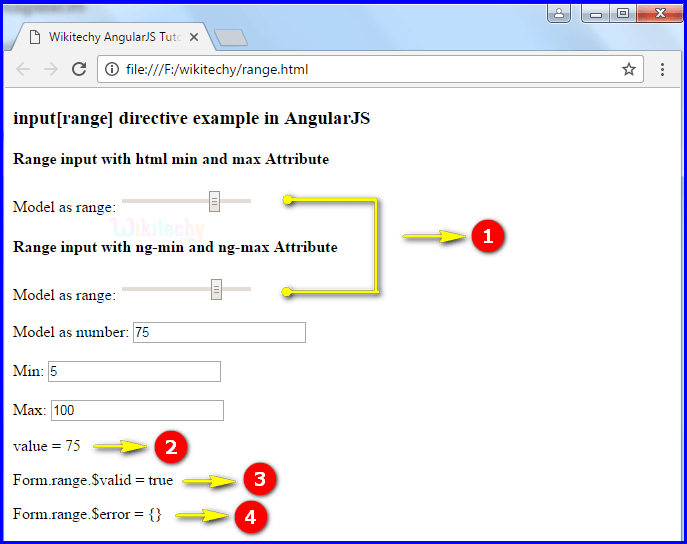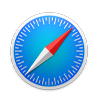# AngularJS Input Range

• input [range] is one of the AngularJS input directive in module ng.
• AnguarJS directive input [range] is used to create an HTML range input with validation and transformation.
• The model for the range input should be a Number.

## Some following values for Angular:

• As the element value must reflect the current model value, a range input will set the ngModel expression to the value that the browser to be set for the input element.
• For ex, <input type=”range” ng-model=”model.value”>
• If sets the value for model.value=null, the browser will set the input to ‘50’ as well as angular will set the model to 50.
• It means the model for range will directly set to 50 after ngmodel has been initialized.
• It does not affect the changes to the model value.
• This directive executes at priority level 0.

## Parameter Values:

Parameter Type Description
ngModel String Defines angular expression to data-bind to.
name (optional) String Name of the form under which the control is available
min(optional) String To set the min validation to ensure that the entered is greater than min.
max(optional) String To set the max validation to ensure that the value entered is shorter than max.
step(optional) String To set the step validation to ensure that the value entered is matches the step.
ngChange(optional) String An expression of Angular to be executed when input changes due to user interaction with the input element.
ngChecked(optional) expression Checked attribute will be set on the element when the expression is truthy.

## Code Explanation input [range] directive in AngularJS:1. The ng-app specifies the root element (“myApp”) to define AngularJS application.
2. ng-controller specifies the application controller in AngularJS the controller value is given as “rangeCtrl”.
3. “range” is declare the type value of the <input> tag.
4. min parameter is used to declare the min value in expression.({{min}})
5. max parameter is used to declare the max value in expression.({{max}})
6. “range” is declare the type value of the <input> tag.
7. ng-min is used to declare the min value in AngularJS.(“min”)
8. ng-max is used to declare the max value in AngularJS(“max”)
9. “number” is declare the type value of the <input> tag.
10. The ng-model bind an input field value to AngularJS application variable (“min”).
11. The ng-model bind an input field value to AngularJS application variable (“max”).
12. Form.range.\$valid to checks the correct range format or not. If the user set the range in the input field then the output will be displays as true otherwise false.
13. Form.range.\$error to check whether the valid range format or not .If the range specified in error it throw an exception (Like “required”=”true) otherwise it is an empty curly braces ( { } ).
14. The values are given as value, min and max in the scope object.

## Sample Output input [range] directive in AngularJS:1. If the user set the range in the input field.
2. The output displays as value=75.
3. The output displays true because it is consider as a valid range value.
4. The output displays empty curly braces it means does not thrown any error.

## Browser Support for input [range] directive in AngularJS:Yes No Yes Yes Yes

## Tips and Notes

• input [range] does not set the min and max attributes, because it is not compatible with ngMax, ngMin and ngStep.
• It means the browser will not automatically adjust the input value , and will always assume min=0, max=100 and step=1